# 基于深度学习的人脸识别系统系列（Caffe+OpenCV+Dlib）——【四】使用CUBLAS加速计算人脸向量的余弦距离

## 前言

CPU：intel i5-4590
GPU：GTX 980

OpenCV版本：3.1（这个无所谓）
Caffe版本：Microsoft caffe （微软编译的Caffe，安装方便，在这里安利一波）
Dlib版本：19.0（也无所谓
CUDA版本：7.5
cudnn版本：4
libfacedetection：6月份之后的（这个有所谓，6月后出了64位版本的）

====================================================================

## 思路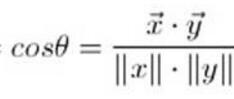x,y均为同维度的向量，如果为6400维，那么我们可以将式子进行展开：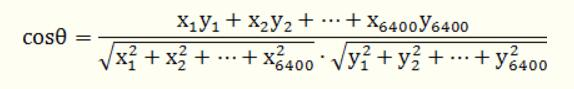## 实现

ComputeDistance.h：

#include <vector>
#include <assert.h>
float cosine(const vector<float>& v1, const vector<float>& v2);

ComputeDistance.cpp：（提供一个总的接口）

#include <ComputeDistance.h>
using namespace std;

float dotProduct(const vector<float>& v1, const vector<float>& v2)
{
assert(v1.size() == v2.size());
float ret = 0.0;
for (vector<float>::size_type i = 0; i != v1.size(); ++i)
{
ret += v1[i] * v2[i];
}
return ret;
}
float module(const vector<float>& v)
{
float ret = 0.0;
for (vector<float>::size_type i = 0; i != v.size(); ++i)
{
ret += v[i] * v[i];
}
return sqrt(ret);
}
float cosine(const vector<float>& v1, const vector<float>& v2)
{
assert(v1.size() == v2.size());
return dotProduct(v1, v2) / (module(v1) * module(v2));
}


main函数：

    Caffe_Predefine();
resize(lena, lena, Size(224, 224));
resize(test, test, Size(224, 224));
if (!lena.empty()&&!test.empty())
{
vector<float> lena_vector = ExtractFeature(lena);
vector<float> test_vector = ExtractFeature(test);
clock_t t1, t2;
t1 = clock();
cout << "余弦距离为：" << cosine(lena_vector, test_vector) << endl;
t2 = clock();
cout << "计算耗时" << t2 - t1 << "ms" << endl;
}
imshow("LENA", lena);
imshow("TEST", test);
waitKey(0);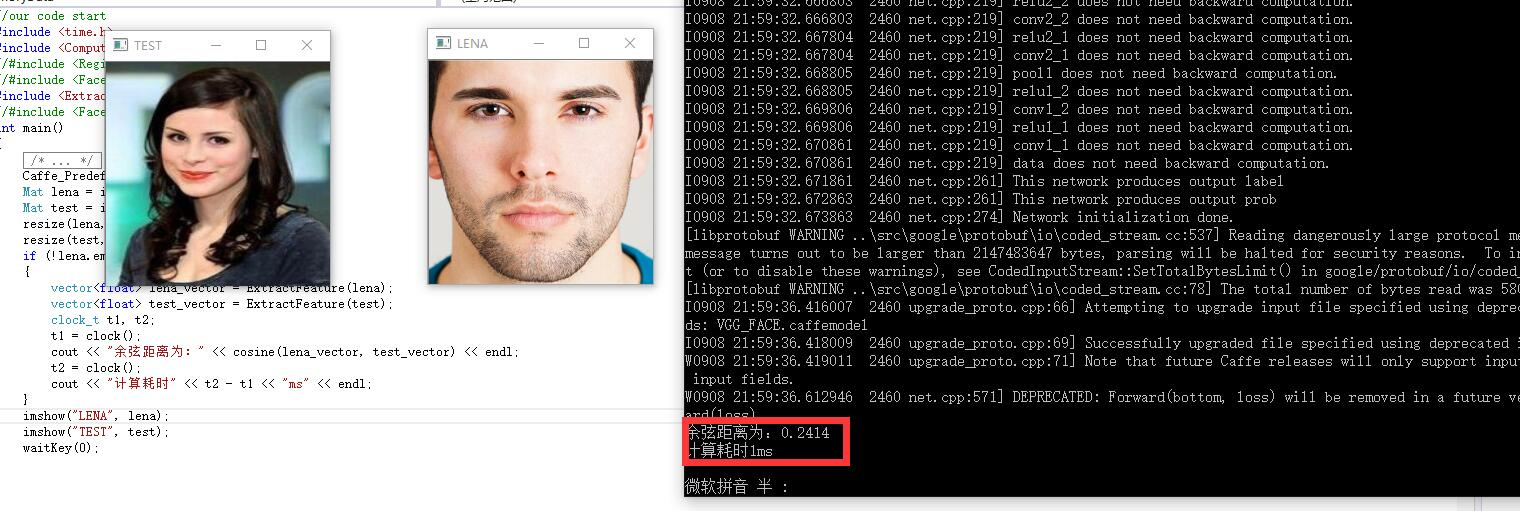## CUBLAS

cublas是一个开源的矩阵加速运算库，它使用更为优秀的浮点运算设备GPU来对线性运算进行加速。因为余弦公式（上面有），我们需要做的核心数学公式及为向量点乘（向量的模可以看成是向量自己对自己的点乘然后再开方）：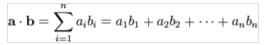cublasSdot_v2 (cublasHandle_t handle,int n,const float *x,int incx,const float *y,int incy,float *result);

n代表的是输入向量的维度，x是第一个向量，y是第二个向量，incx 与incy 取1，result 是点积结果。

#include <stdlib.h>
#include <time.h>
#include <iostream>
#include "cuda_runtime.h"
#include "cublas_v2.h"
using namespace std;
cublasStatus_t ret;
cublasHandle_t handle_cos;

float Cosine(float* a, float* b, float *result,float *a_result,float *b_result,int channel)
{

cublasSdot(handle_cos, channel, a, 1, b, 1, result);
cublasSdot(handle_cos, channel, a, 1, a, 1, a_result);
cublasSdot(handle_cos, channel, b, 1, b, 1, b_result);

return *result/(sqrt(*b_result)*sqrt(*a_result));
}

int main()
{

int arraySize = 10000;
float* a = (float*)malloc(sizeof(float) * arraySize);

float* d_a;
cudaMalloc((void**)&d_a, sizeof(float) * arraySize);

for (int i = 0; i<arraySize; i++)
a[i] = 1.0f;
cudaMemcpy(d_a, a, sizeof(float) * arraySize, cudaMemcpyHostToDevice);
float* result = (float*)malloc(sizeof(float));
float* a_result = (float*)malloc(sizeof(float));
float* b_result = (float*)malloc(sizeof(float));
ret = cublasCreate(&handle_cos);
clock_t t1, t2;
t1 = clock();
cout << Cosine(d_a, d_a, result, a_result, b_result, arraySize) << endl;
t2 = clock();
cout << t2 - t1<<"ms"<<endl;

//printf("\n\nCUBLAS: %.3f", *cb_result);

cublasDestroy(handle_cos);
cin.get();
}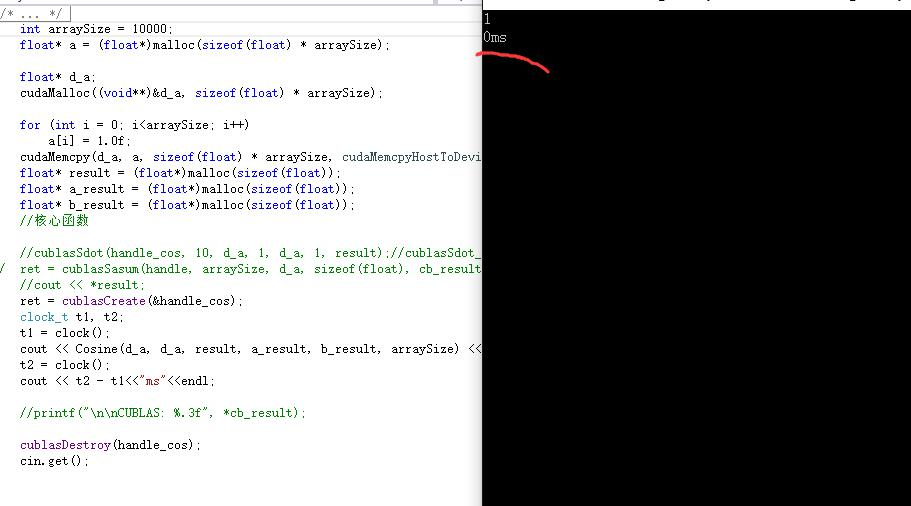=================================================================

### 基于深度学习的人脸识别系统系列（Caffe+OpenCV+Dlib）——【四】使用CUBLAS加速计算人脸向量的余弦距离 完结，如果在代码过程中出现了任何问题，直接在博客下留言即可，共同交流学习。

©️2019 CSDN 皮肤主题: 精致技术 设计师: CSDN官方博客